Courses

# NCERT Solutions - Market Equilibrium Commerce Notes | EduRev

## Commerce : NCERT Solutions - Market Equilibrium Commerce Notes | EduRev

The document NCERT Solutions - Market Equilibrium Commerce Notes | EduRev is a part of the Commerce Course NCERT Textbooks (Class 6 to Class 12).
All you need of Commerce at this link: Commerce

Page No. 84

Question 1: Explain market equilibrium.
Answer: Market equilibrium is defined as the state of rest that is determined by the rational objectives of the consumers and the producers (i.e. maximisation of satisfaction and profit respectively). It is a state where the aggregate quantity that all the firms want to sell are purchased by consumers, i.e. market supply equals market demand. At this situation, there is no incentive or tendency for any change in quantity demanded, quantity supplied and price. That is: yd = ys.

Question 2: When do we say that there is an excess demand for a commodity in the market?
Answer: When the market demand exceeds the market supply at a particular price, then the situation that arises is excess demand. In other words, if at any price, the producers are willing to supply comparatively less than what is demanded by all the consumers in the market, then we face the situation of excess demand.

Question 3: When do we say that there is an excess supply for a commodity in the market?
Answer: Excess supply is a situation when the supply of a commodity in the market exceeds its demand at a particular price. In other words, if at any price level, all the consumers demand comparatively less quantity than what is being supplied by all the suppliers, then we face the situation of excess supply.

Question 4: What will happen if the price prevailing in the market is
(i) above the equilibrium price?
(ii) below the equilibrium price?
Answer: (i) If the market price is above the equilibrium price, there occurs the situation
of excess supply.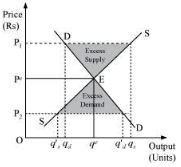In the given figure, the equilibrium price and quantity is demoted by Pe and qe.
Let us assume that the market price (P1) is above the equilibrium price Pe. Now, according to the demand curve, the quantity demanded is qd. Whereas, according to the supply curve, the quantity supplied is qs. Thus, there exists a situation of excess supply equivalent to (qs − qd).
(ii) If the market price is below the equilibrium price, there occurs the situation of excess demand.
Let us assume that the market price P2 is below the equilibrium price Pe. According to the demand curve, quantity demanded is q′d. Whereas, according to the supply curve, the quantity supplied is q′s. So, it can be seen that there emerges the situation of excess supply equivalent to (q′d − q′s).

Question 5: Explain how price is determined in a perfectly competitive market with fixed number of firms.
Answer: When the number of firms in a perfectly competitive market is fixed, the firms are operating in the short-run. The equilibrium price is determined by the intersection of market demand curve and supply curve. It is the price at which the market demand equals market supply.
In the given figure, if at any price above Pe, let us say Rs 12, there will be an excess supply, which will increase the competition among the sellers and they will reduce the price in order to sell more output. This causes a fall in the price, finally to Rs 8 (Pe), where the demand equals supply.
If at any price lower than Pe , let us say Rs 2, there will be an excess demand that will raise the competition among the buyers or consumers and they will be ready to pay higher price for the given output. This will increase the price to Rs 8 (equilibrium price), where the market will reach the equilibrium.
Thus, the invisible hands of market operate automatically whenever there exist excess demand and excess supply; ensuring equilibrium in the market.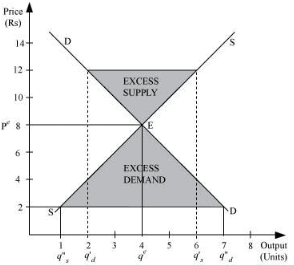Question 6: Suppose the price at which the equilibrium is attained in exercise 5 is above the minimum average cost of the firms constituting the market. Now if we allow for free entry and exit of firms, how will the market price adjust to it?
Answer: If the equilibrium price (Rs 8) in the above figure (of Q-5) is above the minimum of average cost, then it implies that the firm is earning supernormal profits. This situation will attract new firms in the market. As the new firms enter, the industry supply of output will also increase. New firms will continue to enter the industry that will lead the price to fall until it becomes equal to the minimum of the average cost. Thus, the supernormal profits are wiped out and all the firms earn normal profits.
When the free entry and exit of firms is allowed, the equilibrium is determined by the intersection of demand curve and the ‘P = min AC’ line.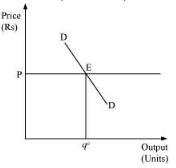Question 7: At what level of price do the firms in a perfectly competitive market supply when free entry and exit is allowed in the market? How is the equilibrium quantity determined in such a market?
Answer: In the long run, due to the free entry and exit of firms, all the firms earn zero economic profit or normal profit. They neither earn abnormal profits nor abnormal losses. Thus, the free entry and exit feature ensures that in the long run the equilibrium price will be equal to the minimum of average cost, irrespective of whether profits or losses are earned in the short run.
The equilibrium is determined by the intersection of consumers’ demand curve and the ‘P = min AC’ line. At equilibrium point E, quantity supplied by each firm is qe at the price (P).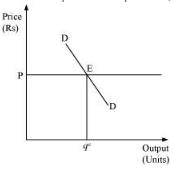Question 8: How is the equilibrium number of firms determined in a market where entry and exist is permitted?
Answer: The characteristic of free entry and exit of firms ensures that all the firms in a perfect competitive market earn normal profit, i.e. the market price is always equal to the minimum of LAC. No new firm will be attracted to enter the market or no existing firm will leave, if the price is equal to the minimum of LAC. Thus, the number of firms is determined by the equality of price and the minimum of LAC. The market equilibrium is determined by the intersection of market demand curve (D1D1) and the price line. The equilibrium price is P1 and the equilibrium output is q1. At this equilibrium price, each firm supplies the same output q1f , as it is assumed that all the firms are identical. Therefore, at the equilibrium, the number of firms in the market is equal to the number of firms required to supply output q1 at price P1, and each in turn supplying q1f amount at this price. That is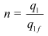Where,
n = number of firms at market equilibrium
q1 = the equilibrium quantity demanded
q1f = the quantity of output supplied by each firm

Question 9: How are equilibrium price and quantity affected when income of the consumers
(a) increase?
(b) decrease?

(a) Increase in income of consumers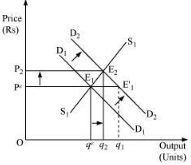If the number of firms is assumed to be fixed, then the increase in consumers’ income will lead the equilibrium price to rise.
Let us understand how it happens:
D1D1 and S1S1 represent the market demand and market supply respectively. The initial equilibrium occurs at E1, where the demand and the supply intersect each other. Due to the increase in consumers’ income, the demand curve will shift rightward parallelly while the supply curve will remain unchanged. Hence, there will be a situation of excess demand, equivalent to (qe − q1). Consequently, the price will rise due to excess demand. The price will continue to rise until it reaches E2 (new equilibrium), where D2D2 intersects the supply curve S1S1. The equilibrium price increases from Pe to P2 and the equilibrium output increases from qe to q2.
(b) Decrease in the income of consumers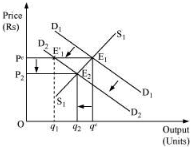The decrease in consumers’ income is depicted by leftward parallel shift of demand curve from D1D1 to D2D2. Consequently, at the price Pe, there will be an execs supply (qe − q1), resulting the price to fall. At the new equilibrium (E2), where D2D2 intersect the supply curve, the equilibrium price falls from Pe to P2 and the equilibrium quantity falls from qe to q2.

Question 10: Using supply and demand curves, show how an increase in the price of shoes affects the price of a pair of socks and the number of pairs of socks bought and sold.
Answer: Shoes and socks both are complementary to each other and are used together. Therefore, the increase in shoe price will discourage the demand for socks. Therefore, due to the decrease in demand for socks, the demand curve for socks will shift leftwards parallelly from D1D1 to D2D2. The supply remaining unchanged, at the equilibrium price Pe, there exists excess supply of socks, which reduces the price of socks and the new equilibrium will be at E2, with equilibrium price P2 and equilibrium quantity q2.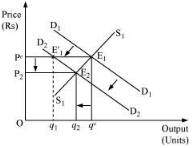Page No 85:

Question 11: How will a change in price of coffee affect the equilibrium price of tea? Explain the effect on equilibrium quantity also through a diagram.
Answer: Coffee and tea are substitute goods, i.e. they are used in the place of each other. An increase or a decrease in the price of coffee will lead to an increase or a decrease in the demand for tea respectively.
The figure depicts the equilibrium of the tea market. The initial demand and supply of tea is depicted by D1D1 and S1S1 respectively. The initial equilibrium is at E1, with the equilibrium price (Pe) and equilibrium quantity (qe). Now, if the price of coffee increases, which will lead to an increase in the demand for tea (being a substitute good), the demand curve of tea will shift rightward parallelly. At the equilibrium price (Pe), there will be an excess demand for tea; consequently, the price of tea will rise. This will form the new equilibrium at E2, with the new equilibrium price P2 and the new equilibrium output q2. Hence, an increase in the price of coffee, will lead the equilibrium price of tea to rise (due to excess demand). Further, the increase in the price of coffee will also lead to the increase in demand for tea as tea is the substitute good for coffee.
Now, if the price of coffee decreases, there will be a decrease in the demand for tea. The demand curve for tea will shift leftward parallelly to D2D2. At the equilibrium price (Pe), there will be an excess supply. Consequently, the price of tea will fall, which will form the new equilibrium at E2, with the new equilibrium price P2 and the new equilibrium output q2. Hence, a decrease in the price of coffee will lead to a decrease in the price of tea and a decrease in the demand for tea, as people will switch over to consumption of coffee.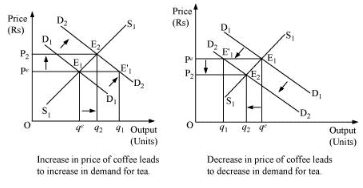Question 12: How do the equilibrium price and the quantity of a commodity change when price of input used in its production changes?
Answer: The change in the price of input alters the cost of production of a commodity.
Let us analyze the two different cases.
1. Increase in input price
If the input price of a firm increases, the cost of production will also increase, which will discourage the firm’s incentive to produce and supply the commodity. This will lead to a left upward shift of the marginal cost curve, which further will lead to a leftward parallel shift of an individual firm’s supply curve and finally a leftward shift of the market supply curve. The demand curve remaining the same, the new equilibrium will occur at E2 with higher equilibrium price (P2) and lower quantity of output (q2).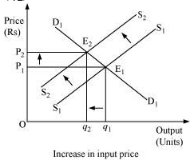2. Decrease in input price
If an input price of a firm decreases, then the cost of production will also decrease. This will shift the marginal cost curve rightward, which implies that the firm’s supply curve will also shift rightward. Consequently, the market supply curve will shift rightward parallelly from S1S1 to S2S2. Demand curve remaining the same, the new equilibrium will occur at E2 with lower equilibrium price (P2) and higher quantity level of output (q2).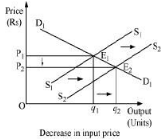Question 13: If the price of a substitute Y of good X increases, what impact does it have on the equilibrium price and quantity of good X?
Answer: X and Y being substitute goods, if the price of Y increases, then it will reduce the demand for Y and people will switch to X, which will raise the demand for X. Thus, the demand curve will shift from D1D1 to D2D2 . At the existing price P1, there will be an excess demand. Due to the pressure of excess demand, the existing price will increase. Consequently, the new equilibrium occurs at E2, where the new demand curve D2D2 intersects the supply curve S1S1. The new equilibrium price is P2, which is higher than P1 and equilibrium quantity is q2, which is higher than q1. Therefore, due to the increase in the price of substitute good Y, the equilibrium price of X will rise and equilibrium output of X will also be higher.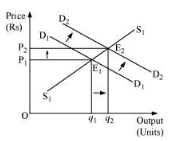Question 14: Compare the effect of shift in the demand curve on the equilibrium when the number of firms in the market is fixed with the situation when entry-exit is permitted.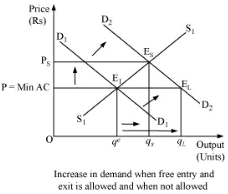The above figure depicts the cases when the number of firms is fixed (in the short run) and when the number of firms is not fixed (in the long run). ‘P = min AC’ represents the long run price line, D1D1 and D2D2 represent the demands in the short run and the long run. The point E1 represents the initial equilibrium where the demand curve and the supply curve intersect each other. Now, let us suppose that the demand curve shifts under the assumption that the number of firms are fixed; thus, the new equilibrium will be at ES (in the short run), where the supply curve S1S1 and the new demand curve D2D2 intersect each other. The equilibrium price is Ps and equilibrium quantity is qs.
Now let us analyse the situation under the assumption of free entry and exit.
The increase in demand will shift the demand curve rightwards to D2D2. The new equilibrium will be at E2. It is the long run equilibr0ium with equilibrium price (P) = min AC and equilibrium quantity qL.
Therefore, on comparing both the cases, we find that when the firms are given the freedom of entry and exit, the equilibrium price remains same and the price is lower than the short run equilibrium price (Ps); whereas, the long run equilibrium quantity (qL) is more than that of the short run equilibrium (qs).
Similarly, for leftward demand shift, it can be noted that the short run equilibrium price (Ps) is less than the long run equilibrium price and the short run equilibrium quantity (qs) is less than the long run equilibrium quantity qL.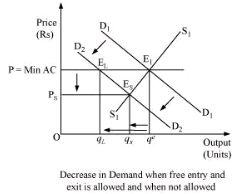Question 15: Explain through a diagram the effect of a rightward shift of both the demand and supply curves on equilibrium price and quantity.
Answer: (a) When demand and supply increase in the same proportion: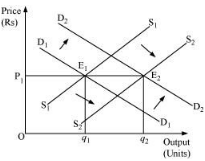E1 is the initial equilibrium with equilibrium price P1 and equilibrium output q1.
Now, let us suppose that the demand increases to D2D2 and the supply increase to S2S2 by the same proportion. The new demand and new supply curve intersect at E2, which is the new equilibrium, with a new equilibrium output q2, but the same equilibrium price P1. Thus, an increase in the demand and the supply by the same proportion leaves the equilibrium price unchanged.
(b) When demand increases more than the increase in supply:
The original demand and supply curves intersect each other at E1 with initial equilibrium price P1 and initial equilibrium output q1.
Now, let us suppose that the demand increases and thereby the demand curve shifts to D2D2; the supply curve also shifts rightwards to S2S2. However, the increase in supply is less than the increase in demand. The new supply curve and the new demand curve intersect each other at point E2 with higher equilibrium price P2 and higher equilibrium output q2.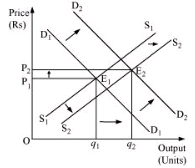(c) When the increase in demand is less than the increase in supply:
Let the initial equilibrium be at E1 with the equilibrium price P1 and equilibrium output q1. Now, let us suppose that the demand increases to D2D2 and the supply increases to S2S2; where the increase in supply is more than that of demand. The new demand curve D2D2 and the new supply curve S2S2 intersect at E2. Thus, the greater increase in supply curve as compared to the demand curve will lead the equilibrium price to fall and equilibrium output to rise.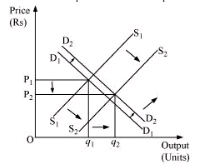Question 16: How are the equilibrium price and quantity affected when
(a) both demand and supply curves shift in the same directions?
(b) demand and supply curves shift in opposite directions?
Answer: (a) both demand and supply curves shift in the same direction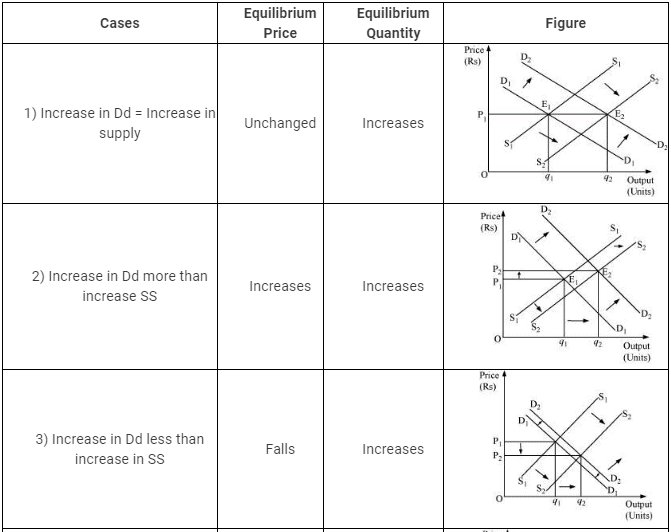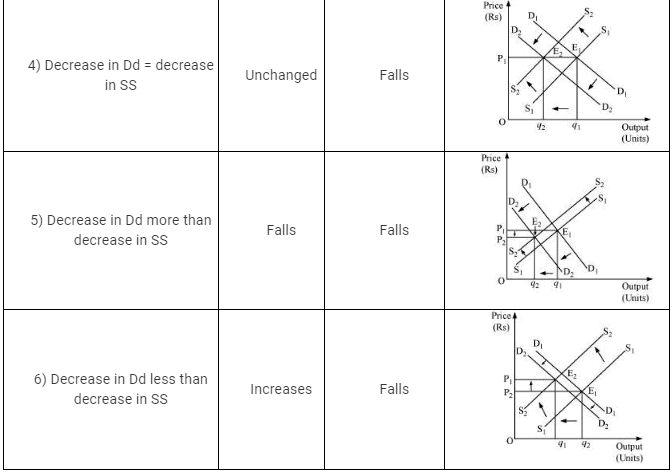(b) demand and supply curves shift in opposite direction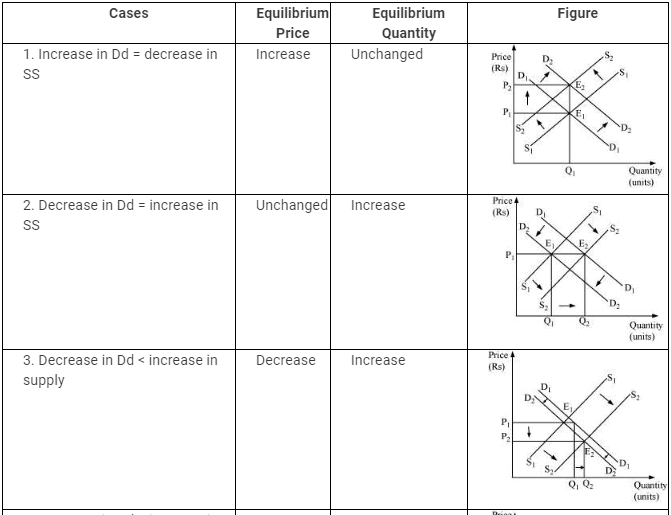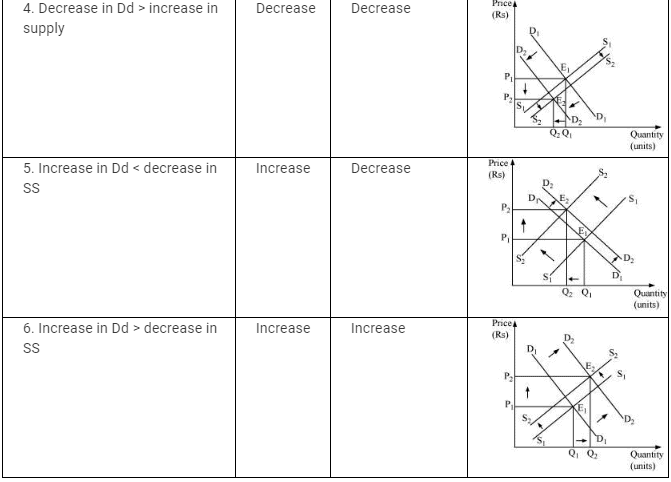Question 17: In what respect do the supply and demand curves in the labour market differ from those in the goods market?
Answer: The supply and demand curves in the labour market differ from those in the goods market in the following ways:
1) In a goods market, the demand for goods is made by consumers or households; while in a labour market, the demand for labour is made by firms.
2) In a goods market, the supply of goods is made by firms; while in a labour market, the supply of labour is made by households.
So, in a goods market, firms act as suppliers; in a labour market, households act as suppliers.

Question 18. How is the optimal amount of labour determined in a perfectly competitive market?
Answer: A profit maximising firm will employ labour up to the point where the extra cost incurred by employing the last unit of labour (wage) equals the additional benefit it earns by employing that unit of labour.
That is, Marginal cost of labour = Marginal benefit by labour
Or, Wage rate = Marginal Revenue Product
Or, w = MRPL
Or, w = MR × MPL (as MRPL = MR × MPL)
Or, w = P × MPL (in Perfect competition Price = MR)
Or, w = VMPL (because VMPL = P × MPL)
The demand for labour is derived from VMPL and the supply of labour is positively sloped. The equilibrium exists at E, where the demand for labour and the supply of labour intersect each other. The equilibrium wage rate is w and optimal amount of labour is qL.

Question 19: How is the wage rate determined in a perfectly competitive labour market?
Answer: Similar to a goods market, wage rate in a labour market is determined by the intersection of demand for labour and supply of labour. The rate at which the demand equals the supply is called the equilibrium wage rate. Corresponding hours of labour are demanded and supplied in the labour market at the equilibrium wage rate.
The demand for labour is derived from the value of marginal product of labour (VMPL). We know that a particular firm will employ labour up to a point where marginal cost of employing the last unit of labour hired equals the marginal benefit earned by the firm by hiring that unit of labour.
Labour is supplied by those households, who need to trade-off between working hours (labour) or leisure. The supply of labour is a positive function of wage up to a point beyond which the supply curve becomes backward bending supply curve.
The intersection of demand for labour and the supply of labour occur at the wage rate w. Here, the equilibrium takes place at E where DLDL equals SLSL and the equilibrium units of labour supplied and demanded is L.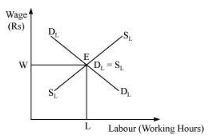Question 20: Can you think of any commodity on which price ceiling is imposed in India? What may be the consequences of price ceiling
Answer: In India, there are many goods on which government has imposed price ceiling, in order to keep them available within the reach of the BPL (below poverty lime) people. These goods are kerosene, sugar, wheat, rice, etc.
The following are the consequences of price ceiling:
1) Excess demand − Due to artificially imposed price, cutting lower than the equilibrium price leads to the emergence of the problem of excess demand.
2) Fixed Quota − Each consumer gets a fixed quantity of good (as per the quota). The quantity often falls short of meeting the individual’s requirements. This further leads to the problem of shortage and the consumer remains unsatisfied.
3) Inferior goods − Often it has been found that the goods that are rationed are usually inferior goods and are adulterated.
4) Black marketing − The needs of a consumer remains unfulfilled as per the quota laid by the government. Consequently, some of the unsatisfied consumers get ready to pay higher price for the additional quantity. This leads to black-marketing and artificial shortage in the market.

Question 21: A shift in demand curve has a larger effect on price and smaller effect on quantity when the number of firms is fixed compared to the situation when free entry and exit is permitted.Explain.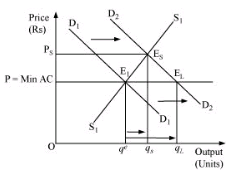The above figure depicts both the cases when the number of firms is fixed (in short run) and when the number of firms is not fixed (in long run). P = min AC represents the long run price line; D1D1 and D2D2 represents the demand in the short run and the long run respectively. The point E1 represents the initial equilibrium, where the demand and the supply intersect each other.
Let us suppose that the demand curve shifts, assuming that the number of firms is fixed. Now, the new equilibrium will be at ES (as it is short run equilibrium), where the supply curve and the demand curve D2D2 intersect each other. The equilibrium price is Ps and equilibrium quantity is qs.
On the other hand, under the assumption of free entry and exit, an increase in demand will shift the demand curve rightwards to D2D2. The new equilibrium will be at E2 (as it is a long run equilibrium) with the equilibrium price P = min AC and equilibrium quantity qL.
Therefore, on comparing both the cases, we find that when the firms are given the freedom of entry and exit, the equilibrium price remains the same. The price is lower than that of the short run equilibrium price (Ps); whereas, the long run equilibrium quantity (qL) is more than that of the short run equilibrium quantity (qs).
Similarly, for the leftward demand shift, it can be found that the short run equilibrium price (Ps) is lower than the long run equilibrium price and the short run equilibrium quantity (qs) in less than the long run equilibrium quantity (qL)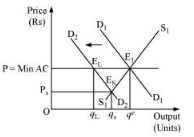Question 22: Suppose the demand and supply curve of commodity X in a perfectly competitive market are given by:
qD = 700 − p
qS = 500 + 3p for p ≥ 15
= 0 or 0 ≤ p 15
Assume that the market consists of identical firms. Identify the reason behind the market supply of commodity X being zero at any price less than Rs 15. What will be the equilibrium price for this commodity? At equilibrium, what quantity of X will be produced?

qd = 700 − p
qs = 500 + 3p for p > Rs 15
= 0 for 0 ≤ p < 15
The market supply is zero for any price from Rs 0 to Rs 15, this is because, for price between 0 to 15, no individual firm will produce any positive level of output (as the price is less than the minimum of AVC). Consequently, the market supply curve will be zero.
At equilibrium qd = qs
700 − p = 500 + 3p
− p −3p = 500 − 700
− 4p = − 200
p = 50
Equilibrium price is Rs 50.
Quantity = qs = 500 + 3p
= 500 + 3 (50)
= 500 + 150
= 650
Therefore, the equilibrium quantity is 650 units.

Question 23: Considering the same demand curve as in exercise 22, now let us allow for free entry and exit of the firms producing commodity X. Also assume the market consists of identical firms producing commodity X. Let the supply curve of a single firm be explained as
qSf = 8 + 3p for p ≥ 20
= 0 for 0 ≤ p < 20
(a) What is the significance of p = 20?
(b) At what price will the market for X be in equilibrium? State the reason for your answer
(c) Calculate the equilibrium quantity and number of firms.

qsf = 8 + 3 p for p ≥ Rs 200
= 0 for 0 ≤ p < Rs 20.
qd = 700 − p
(a) For the price between 0 to 20, no firm is going to produce anything as the price in this range is below the minimum of LAC. So, at the price of Rs 20, the price line is equal to the minimum of LAC.
(b) As there exists the freedom of entry and exit of firms, the minimum of AVC is at Rs 20, also, the price of Rs 20 is the equilibrium price. This is because in the long run, all firms earn zero economic profit, which implies that the price of Rs 20 is the equilibrium price and at any price lower than Rs 20, the firm will move out of the market.
(c) At equilibrium price of Rs 20
Quantity supplied = q= 8 + 3p
= 8 + 3 (20)
qs = 68 units
Quantity demanded qd= 700 − p
= 700 − 20
qd = 680
Number of firms (n)=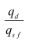n=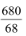n = 10 firms
Therefore, the number of firms in the market is 10 and the equilibrium quantity in 680 units.

Question 24: Suppose the demand and supply curves of salt are given by:
qD = 1,000 − p qS = 700 + 2p
(a) Find the equilibrium price and quantity.
(b) Now, suppose that the price of an input that used to produce salt has increased so, that the new supply curve is
q= 400 +2p
How does the equilibrium price and quantity change? Does the change conform to your expectation?
(c) Suppose the government has imposed a tax of Rs 3 per unit of sale on salt. How does it affect the equilibrium rice quantity?

qd = 1000 − p — (1)
qs = 700 + 2p — (2)
(a) At equilibrium
qd = qs
1000 − p = 700 + 2p
300 = 3 p
100 = p
p = Rs 100
qd = 1000-100 [Substituting the value of p in equation (1)]
= 900 units
So, the equilibrium price is Rs 100 and equilibrium quantity is 900 units.
(b) New quantity supplied (q′s)
q′s = 400 + 2p
At equilibrium qd = q′s
1000 − p = 400 + 2p
600 = 3p
200 = p
p = Rs 200
Prior to the increase in the price of input, the equilibrium price was Rs 100, and after the rise in input’s price, the equilibrium price is Rs 200.
So the change in the equilibrium price in Rs 100 (200 − 100).
qd = 4000 − 200 [Subtitling the value of p in equation (1)]
= 800 units
The change in the equilibrium quantity is 100 units (i.e. 900 − 800 units).
Yes, this change is obvious, as due to the change in the input’s price, the cost of producing salt has increased that will shift the marginal cost curve leftward and move the supply curve to the left. A leftward shift in the supply curve results in a rise in the equilibrium price and a fall in the equilibrium quantity.
(c) The imposition of tax of Rs 3 per unit of salt sold will raise the cost of producing salt. This will shift the supply curve leftwards and the quantity supplied equation will become
ys = 700 + 2 (p − 3)
At equilibrium
yd = ys
1000 − p = 700 +2 (p − 3)
1000 − p = 700 +2p − 6
306 = 3p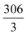= p
p = Rs 102
Substituting the value of p in equation (1)
yd = 1000 − p
yd = 1000 − 102
yd = 898 units
Thus, the imposition of tax of Rs 3 per unit of salt sold will result in an increase in the price of salt from Rs 100 to Rs 102. The equilibrium quantity falls from 900 units to 898 units.

Question 25: Suppose the market determined rent for apartments is too high for common people to afford. If the government comes forward to help those, seeking apartments on rent by imposing control on rent, what impact will it have on the market for apartments?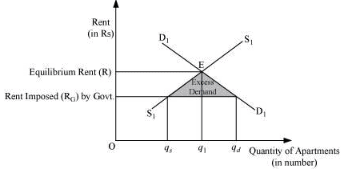The above figure depicts an equilibrium and an effect of price ceiling (maximum rent).
The market demand for apartments is depicted by the D1D1 curve and the supply of apartments is depicted by S1S1. The equilibrium price determined is R and the equilibrium quantity is q.
If the government steps in and imposes rent ceiling (maximum rent) equivalent to RG, then at this rent, there will be an excess demand. The quantity of apartments demanded will be qd. Whereas, the quantity of apartments supplied is qs. So, there exists an excess demand equivalent to qd − qs. At the rate RG, common people can afford apartments to live in, which earlier they were not able to. However, besides this positive effect of imposition of maximum rent, it might happen that some landlords indulge in the practice of black marketing and offer apartments for rent at comparatively higher price.

,

,

,

,

,

,

,

,

,

,

,

,

,

,

,

,

,

,

,

,

,

;